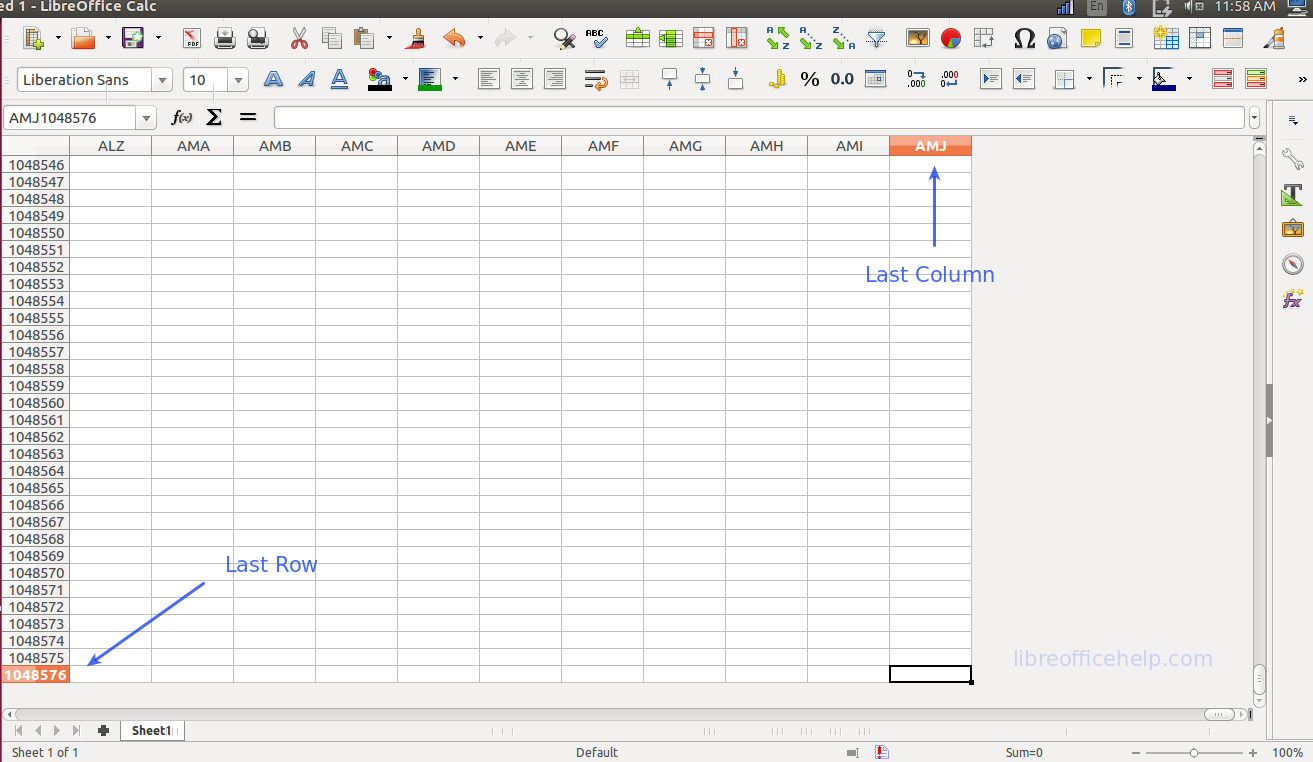# Maximum Number of Rows, Columns, Cells in LibreOffice Calc

By | January 8, 2020

This guide lists the maximum number of rows, columns, and cells in LibreOffice Calc.

LibreOffice Calc has below limits for number of rows, columns and cells that it can handle.

• Maximum number of Columns per worksheet = 1024 (Col A to AMJ).
• Maximum number of Rows per worksheet = 1048576 (2^20).
• Maximum number of cells per worksheet = 1,073,741,824 (2^30).
• Maximum number of characters in one cell = 32767
• Maximum number of worksheets in a workbook = 10000